# Resources tagged with: Addition & subtraction

Filter by: Content type:
Age range:
Challenge level:

### There are 214 results### Exploring Wild & Wonderful Number Patterns

##### Age 7 to 11 Challenge Level:

EWWNP means Exploring Wild and Wonderful Number Patterns Created by Yourself! Investigate what happens if we create number patterns using some simple rules.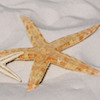### Starfish Spotting

##### Age 5 to 7 Challenge Level:

How many starfish could there be on the beach, and how many children, if I can see 28 arms?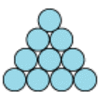### Difference

##### Age 7 to 11 Challenge Level:

Place the numbers 1 to 10 in the circles so that each number is the difference between the two numbers just below it.### What's in a Name?

##### Age 5 to 7 Challenge Level:

What do you notice about these squares of numbers? What is the same? What is different?### Abundant Numbers

##### Age 7 to 11 Challenge Level:

48 is called an abundant number because it is less than the sum of its factors (without itself). Can you find some more abundant numbers?### Calendar Calculations

##### Age 7 to 11 Challenge Level:

Try adding together the dates of all the days in one week. Now multiply the first date by 7 and add 21. Can you explain what happens?### The Deca Tree

##### Age 7 to 11 Challenge Level:

Find out what a Deca Tree is and then work out how many leaves there will be after the woodcutter has cut off a trunk, a branch, a twig and a leaf.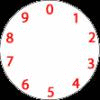### Dividing a Cake

##### Age 7 to 11 Challenge Level:

Annie cut this numbered cake into 3 pieces with 3 cuts so that the numbers on each piece added to the same total. Where were the cuts and what fraction of the whole cake was each piece?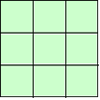### 1, 2, 3 Magic Square

##### Age 7 to 11 Challenge Level:

Arrange three 1s, three 2s and three 3s in this square so that every row, column and diagonal adds to the same total.### The Clockmaker's Birthday Cake

##### Age 7 to 11 Challenge Level:

The clockmaker's wife cut up his birthday cake to look like a clock face. Can you work out who received each piece?### Code Breaker

##### Age 7 to 11 Challenge Level:

This problem is based on a code using two different prime numbers less than 10. You'll need to multiply them together and shift the alphabet forwards by the result. Can you decipher the code?### Sometimes We Lose Things

##### Age 7 to 11 Challenge Level:

Well now, what would happen if we lost all the nines in our number system? Have a go at writing the numbers out in this way and have a look at the multiplications table.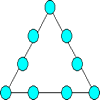### Magic Triangle

##### Age 7 to 11 Challenge Level:

Place the digits 1 to 9 into the circles so that each side of the triangle adds to the same total.### Zios and Zepts

##### Age 7 to 11 Challenge Level:

On the planet Vuv there are two sorts of creatures. The Zios have 3 legs and the Zepts have 7 legs. The great planetary explorer Nico counted 52 legs. How many Zios and how many Zepts were there?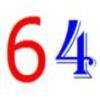##### Age 7 to 11 Challenge Level:

Write the numbers up to 64 in an interesting way so that the shape they make at the end is interesting, different, more exciting ... than just a square.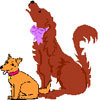### Big Dog, Little Dog

##### Age 5 to 7 Challenge Level:

Woof is a big dog. Yap is a little dog. Emma has 16 dog biscuits to give to the two dogs. She gave Woof 4 more biscuits than Yap. How many biscuits did each dog get?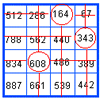### Marvellous Matrix

##### Age 7 to 11 Challenge Level:

Follow the directions for circling numbers in the matrix. Add all the circled numbers together. Note your answer. Try again with a different starting number. What do you notice?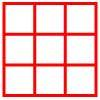##### Age 5 to 11 Challenge Level:

Place six toy ladybirds into the box so that there are two ladybirds in every column and every row.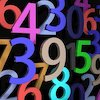### Countdown

##### Age 7 to 14 Challenge Level:

Here is a chance to play a version of the classic Countdown Game.### Got it for Two

##### Age 7 to 14 Challenge Level:

Got It game for an adult and child. How can you play so that you know you will always win?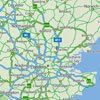### Journeys

##### Age 7 to 11 Challenge Level:

Investigate the different distances of these car journeys and find out how long they take.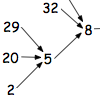##### Age 7 to 11 Challenge Level:

What happens when you add the digits of a number then multiply the result by 2 and you keep doing this? You could try for different numbers and different rules.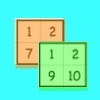### Sorting the Numbers

##### Age 5 to 11 Challenge Level:

Complete these two jigsaws then put one on top of the other. What happens when you add the 'touching' numbers? What happens when you change the position of the jigsaws?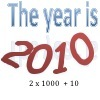### It Was 2010!

##### Age 5 to 11 Challenge Level:

If the answer's 2010, what could the question be?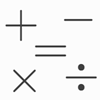### Which Symbol?

##### Age 7 to 11 Challenge Level:

Choose a symbol to put into the number sentence.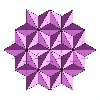### Next Number

##### Age 7 to 11 Short Challenge Level:

Find the next number in this pattern: 3, 7, 19, 55 ...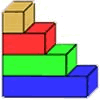### Rod Measures

##### Age 7 to 11 Challenge Level:

Using 3 rods of integer lengths, none longer than 10 units and not using any rod more than once, you can measure all the lengths in whole units from 1 to 10 units. How many ways can you do this?### Month Mania

##### Age 5 to 11 Challenge Level:

Can you design a new shape for the twenty-eight squares and arrange the numbers in a logical way? What patterns do you notice?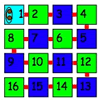### Caterpillars

##### Age 5 to 7 Challenge Level:

These caterpillars have 16 parts. What different shapes do they make if each part lies in the small squares of a 4 by 4 square?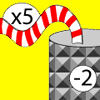### Function Machines

##### Age 7 to 11 Challenge Level:

If the numbers 5, 7 and 4 go into this function machine, what numbers will come out?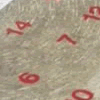### All Seated

##### Age 7 to 11 Challenge Level:

Look carefully at the numbers. What do you notice? Can you make another square using the numbers 1 to 16, that displays the same properties?### Diagonal in a Spiral

##### Age 7 to 11 Challenge Level:

Investigate the totals you get when adding numbers on the diagonal of this pattern in threes.### Street Sequences

##### Age 5 to 11 Challenge Level:

Investigate what happens when you add house numbers along a street in different ways.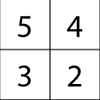### Magic Matrix

##### Age 7 to 11 Challenge Level:

Find out why these matrices are magic. Can you work out how they were made? Can you make your own Magic Matrix?### The Pied Piper of Hamelin

##### Age 7 to 11 Challenge Level:

This problem is based on the story of the Pied Piper of Hamelin. Investigate the different numbers of people and rats there could have been if you know how many legs there are altogether!### Sending Cards

##### Age 7 to 11 Challenge Level:

This challenge asks you to investigate the total number of cards that would be sent if four children send one to all three others. How many would be sent if there were five children? Six?### I'm Eight

##### Age 5 to 11 Challenge Level:

Find a great variety of ways of asking questions which make 8.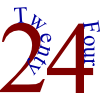### The 24 Game

##### Age 7 to 11 Challenge Level:

There are over sixty different ways of making 24 by adding, subtracting, multiplying and dividing all four numbers 4, 6, 6 and 8 (using each number only once). How many can you find?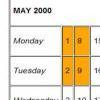### Calendar Patterns

##### Age 7 to 11 Challenge Level:

In this section from a calendar, put a square box around the 1st, 2nd, 8th and 9th. Add all the pairs of numbers. What do you notice about the answers?### Neighbours

##### Age 7 to 11 Challenge Level:

In a square in which the houses are evenly spaced, numbers 3 and 10 are opposite each other. What is the smallest and what is the largest possible number of houses in the square?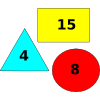### Super Value Shapes

##### Age 7 to 11 Challenge Level:

If each of these three shapes has a value, can you find the totals of the combinations? Perhaps you can use the shapes to make the given totals?### A Square of Numbers

##### Age 7 to 11 Challenge Level:

Can you put the numbers 1 to 8 into the circles so that the four calculations are correct?### First Connect Three

##### Age 7 to 14 Challenge Level:

Add or subtract the two numbers on the spinners and try to complete a row of three. Are there some numbers that are good to aim for?### Sam's Quick Sum

##### Age 7 to 11 Challenge Level:

What is the sum of all the three digit whole numbers?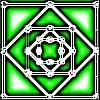### Number Squares

##### Age 5 to 11 Challenge Level:

Start with four numbers at the corners of a square and put the total of two corners in the middle of that side. Keep going... Can you estimate what the size of the last four numbers will be?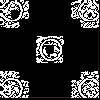### Doplication

##### Age 7 to 11 Challenge Level:

We can arrange dots in a similar way to the 5 on a dice and they usually sit quite well into a rectangular shape. How many altogether in this 3 by 5? What happens for other sizes?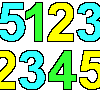### Sept03 Sept03 Sept03

##### Age 7 to 11 Challenge Level:

This number has 903 digits. What is the sum of all 903 digits?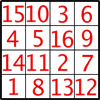### Magic Constants

##### Age 7 to 11 Challenge Level:

In a Magic Square all the rows, columns and diagonals add to the 'Magic Constant'. How would you change the magic constant of this square?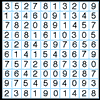### A Numbered Route

##### Age 7 to 11 Challenge Level:

Can you draw a continuous line through 16 numbers on this grid so that the total of the numbers you pass through is as high as possible?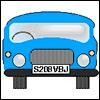### Numbered Cars

##### Age 7 to 11 Challenge Level:

I was looking at the number plate of a car parked outside. Using my special code S208VBJ adds to 65. Can you crack my code and use it to find out what both of these number plates add up to?